Download the eSaral app and start learning from Kota's top IITians and doctors.

# NCERT Solutions for Class 10 Maths chapter 10 Exercise 10.2 - Circles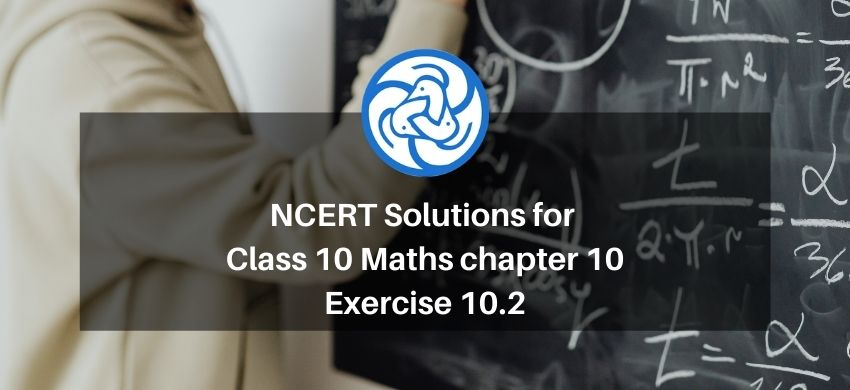Hey, are you a class 10 Student and Looking for Ways to Download NCERT Solutions for Class 10 Maths Chapter 10 Exercise 10.2? If Yes then you are at the right place.

Here we have listed Class 10 Maths Chapter 10 exercise 10.2 solutions in PDF that is prepared by Kota’s top IITian’s Faculties by keeping Simplicity in mind.

If you want to score high in your class 10 Maths Exam then it is very important for you to have a good knowledge of all the important topics, so to learn and practice those topics you can use eSaral NCERT Solutions.

So, without wasting more time Let’s start.

### Download The PDF of NCERT Solutions for Class 10 Maths chapter 10 Exercise 10.2 "Circles"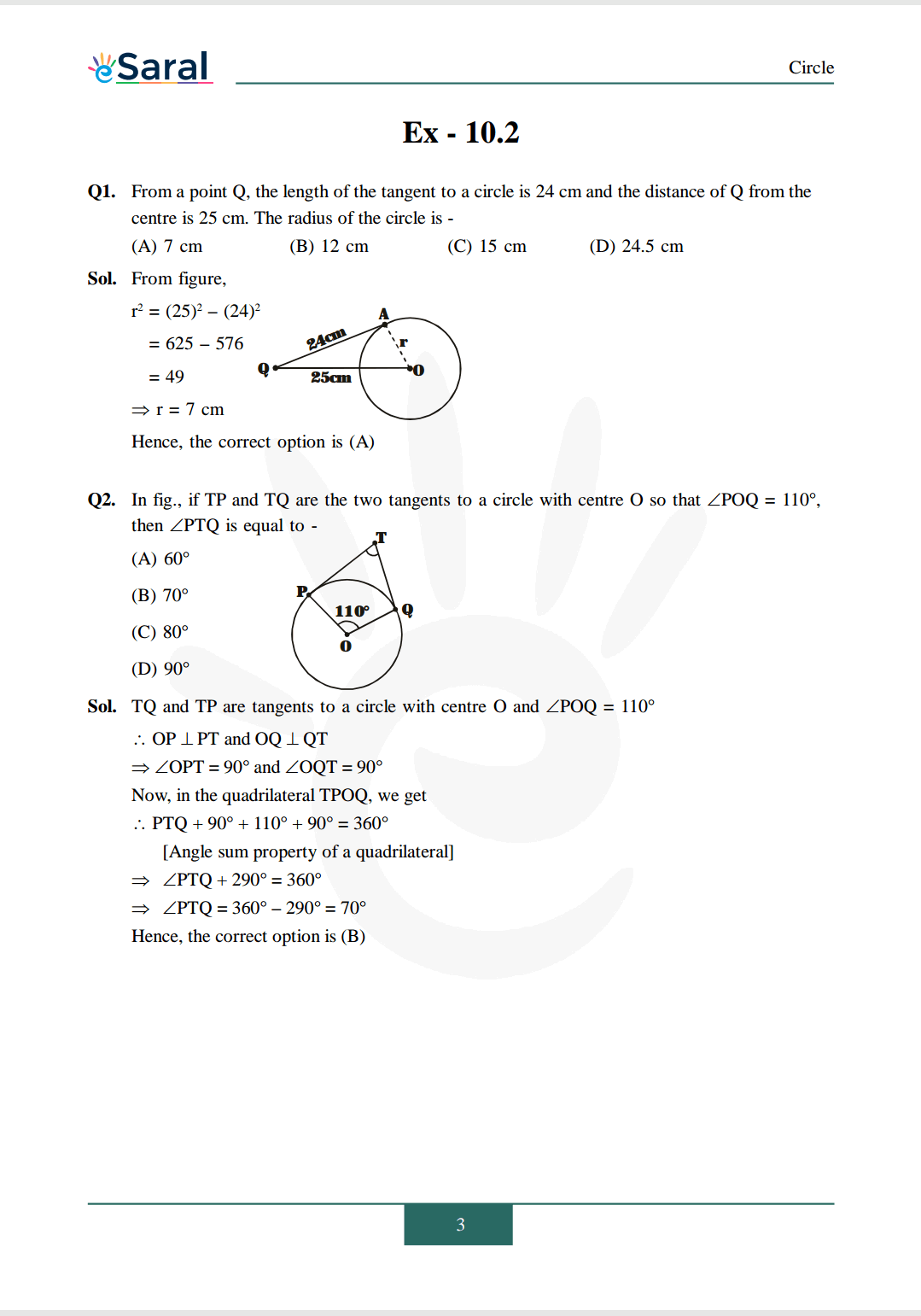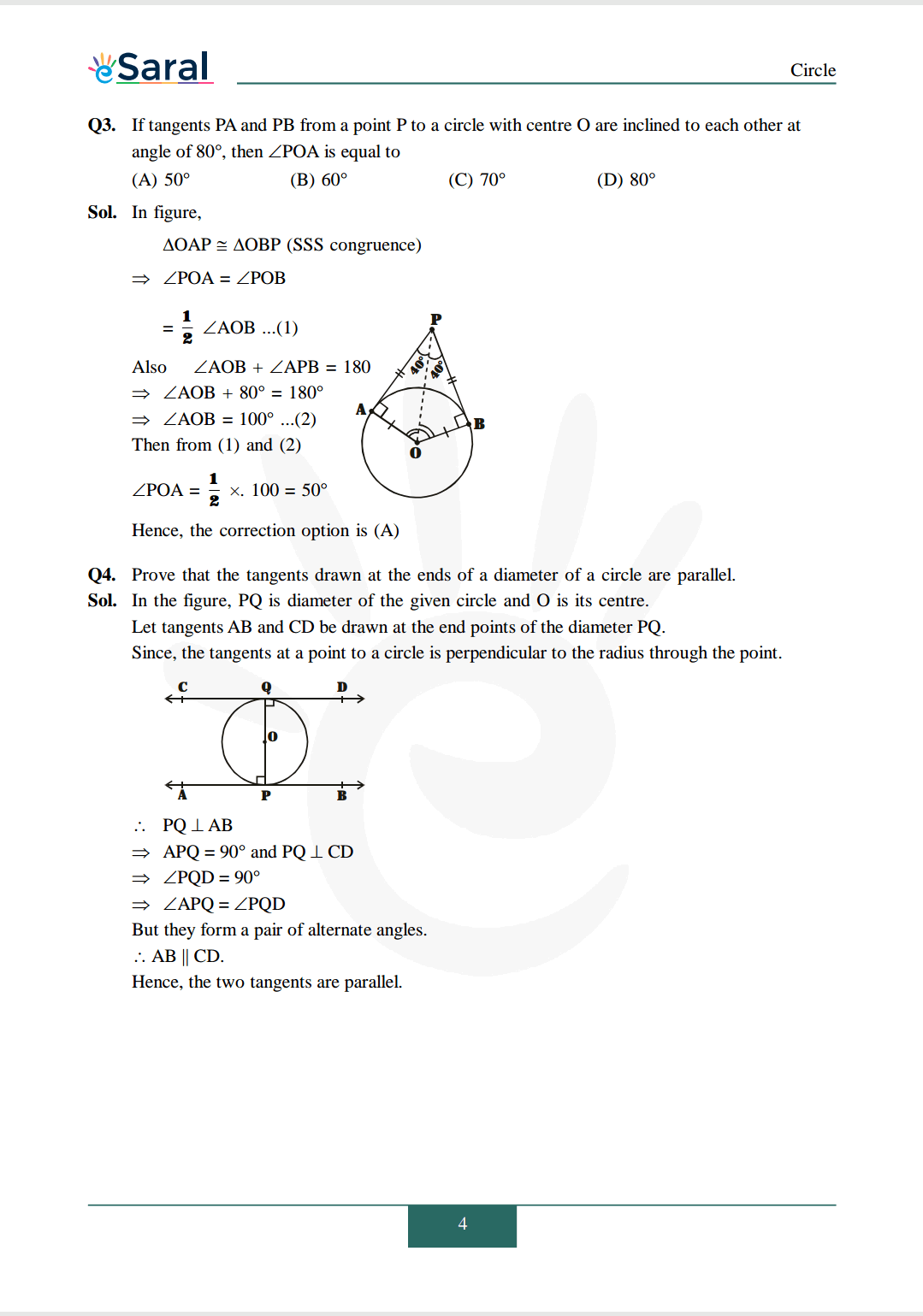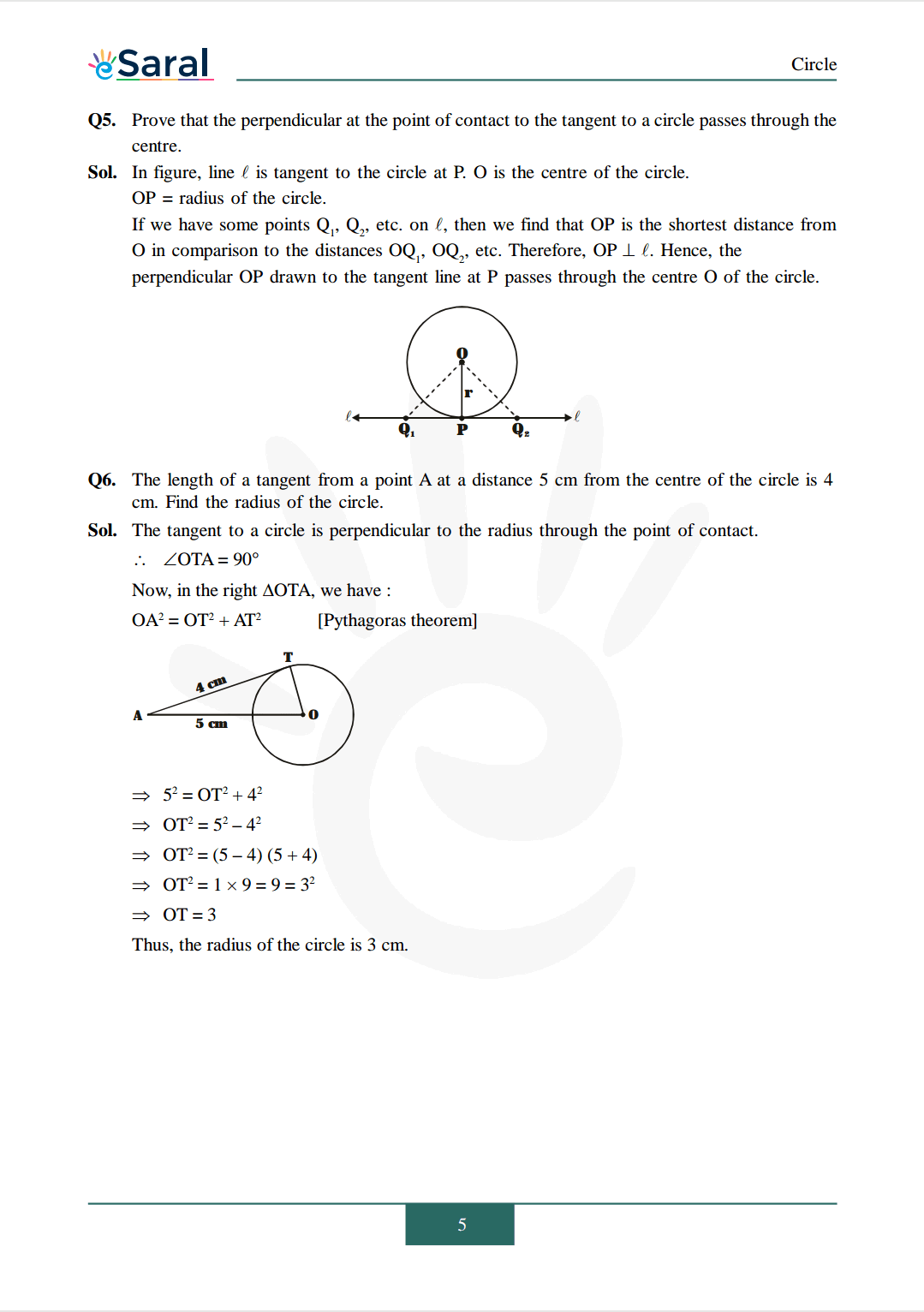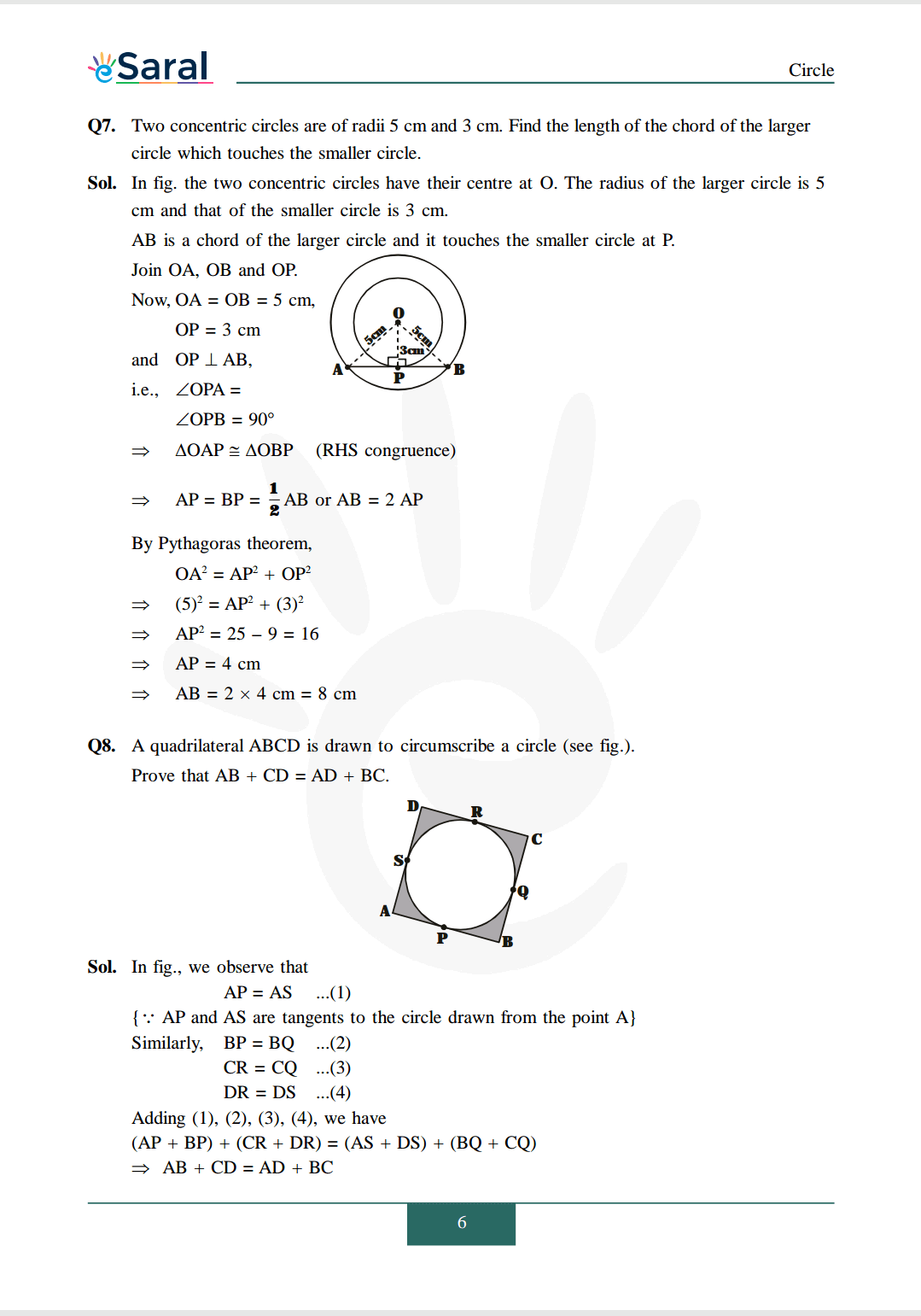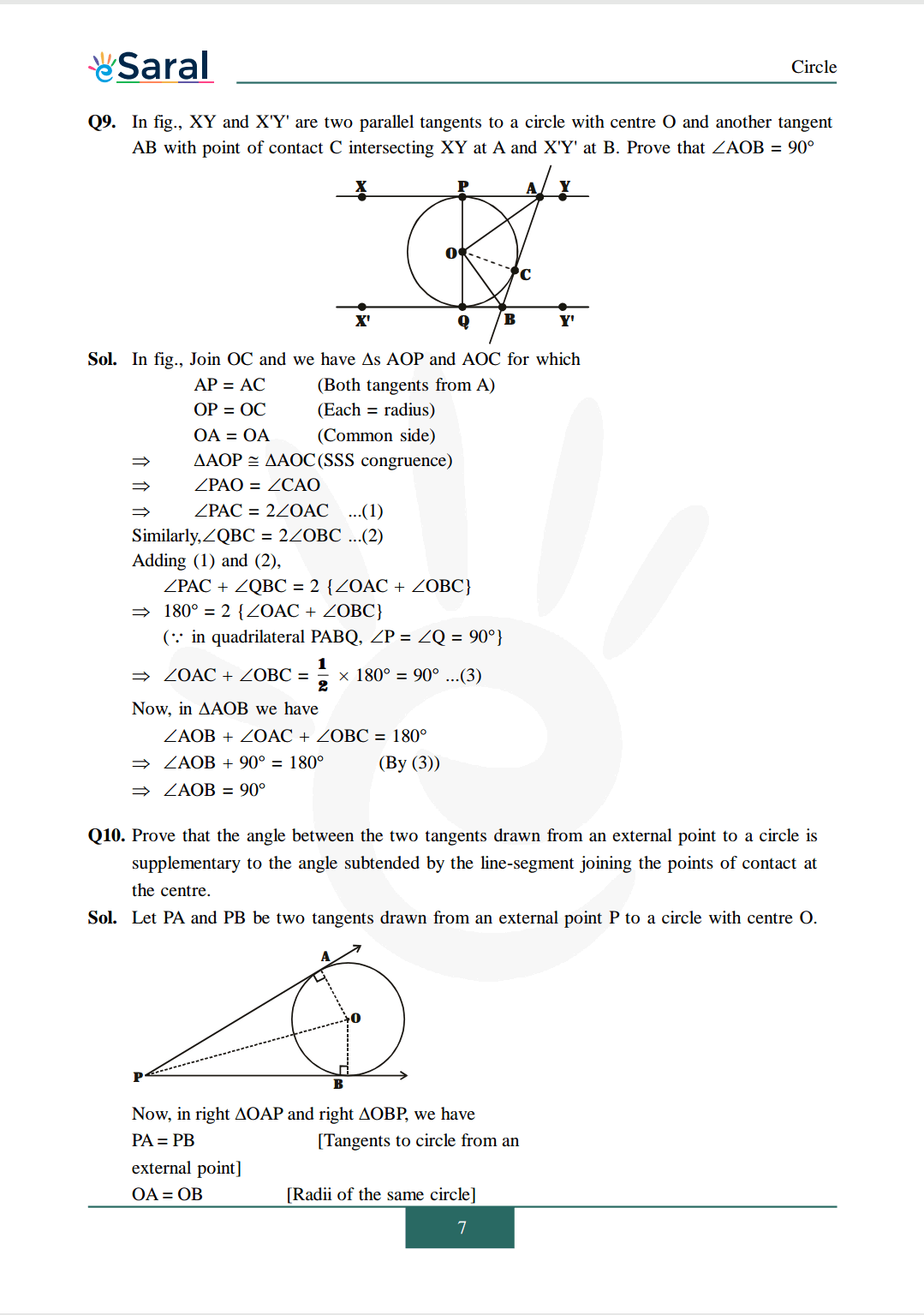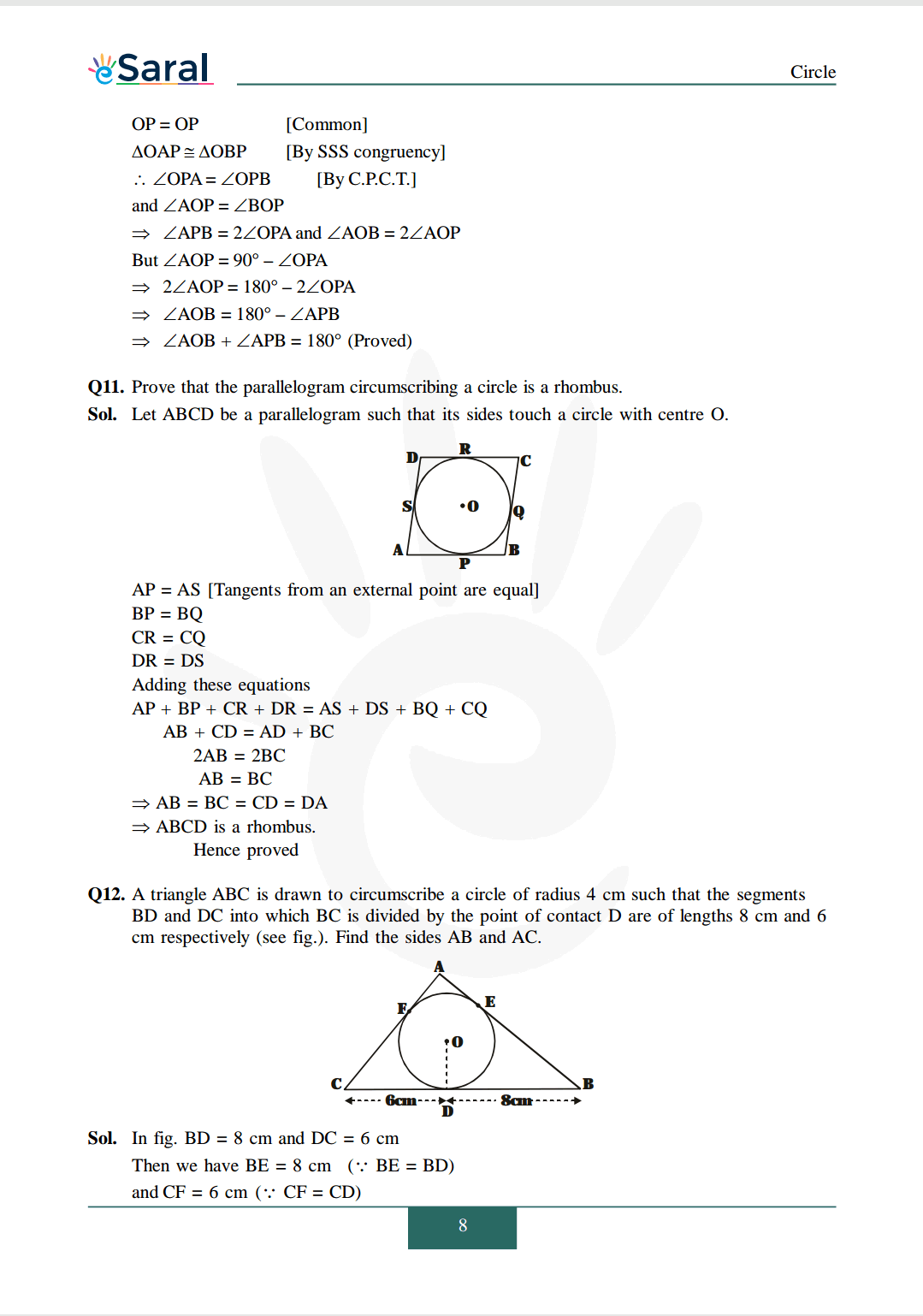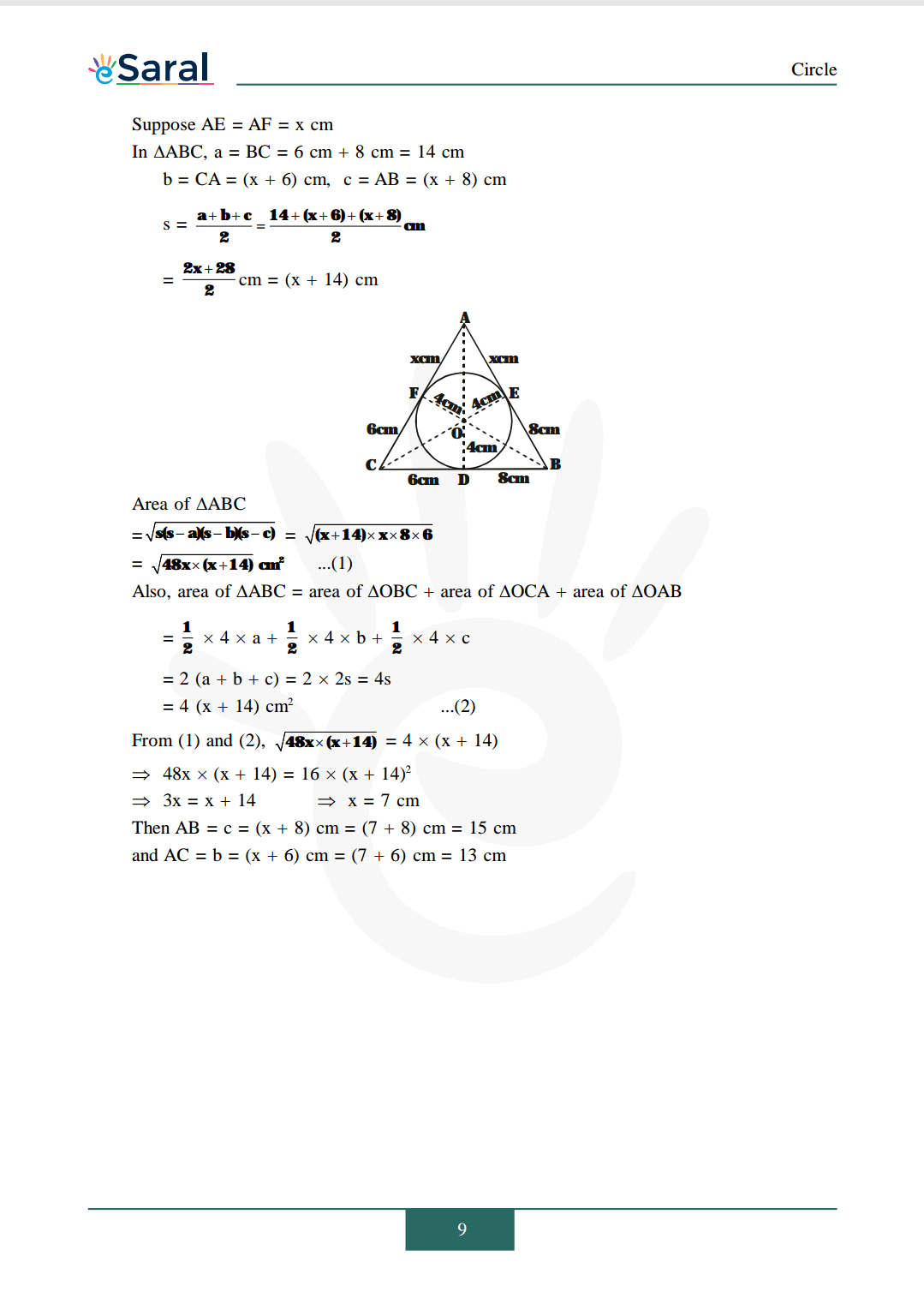NCERT Class 10 Maths Chapterwise Book

NCERT Class 10 Maths Chapterwise Exemplar

Complete Solutions for Class 10 Maths chapter 10

NCERT Class 10 Maths Chapter 8 Exercise 8.1 Solutions

NCERT Class 10 Maths Chapter 8 Exercise 8.2 Solutions

NCERT Class 10 Maths Chapter 8 Exercise 8.3 Solutions

NCERT Class 10 Maths Chapter 8 Exercise 8.4 Solutions

NCERT Class 10 Maths Chapter 9 Exercise 9.1 Solutions

If you have any Confusion related to NCERT Solutions for Class 10 Maths Chapter 10 Exercise 10.2 then feel free to ask in the comments section down below.

To watch Free Learning Videos on Class 10 by Kota’s top Faculties Install the eSaral App